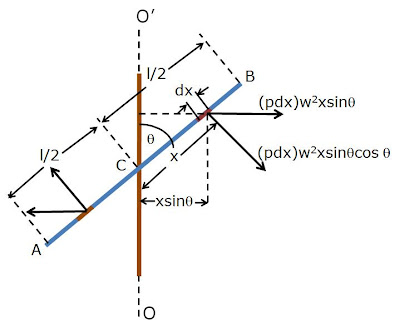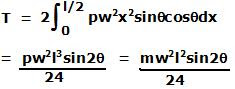## Thursday, February 12, 2009

### Irodov Problem 1.269In this problem as the rod rotates, each point along the rod rotates at a different radius and thus experiences a different centrifugal force. The centrifugal force acts in the radially outward direction and generates a torque on the rod trying to make the rod perpendicular to the axis of rotation.

Consider an infinitesimally small length of the rod of length dx that is at a distance x from the center of the rod O. Let the linear density (mass per unit length) of the rod be p. The mass of this infinitesimally small piece of the rod is pdl. The radius of rotation of this piece of rod, as shown in the figure is. The piece thus, experiences a centrifugal force acting radially outwards of magnitude,The component of this force perpendicular to the length of the rod is responsible for inducing the torque on the rod and is given by,The moment arm of this force on the infinitesimally small piece of rod is x, and so the torque is given by,The net torque acting on the part BC of the rod is exactly equal to that acting on the part AC. The net torque can be found by integrating dT for all x over this part of the rod given by,